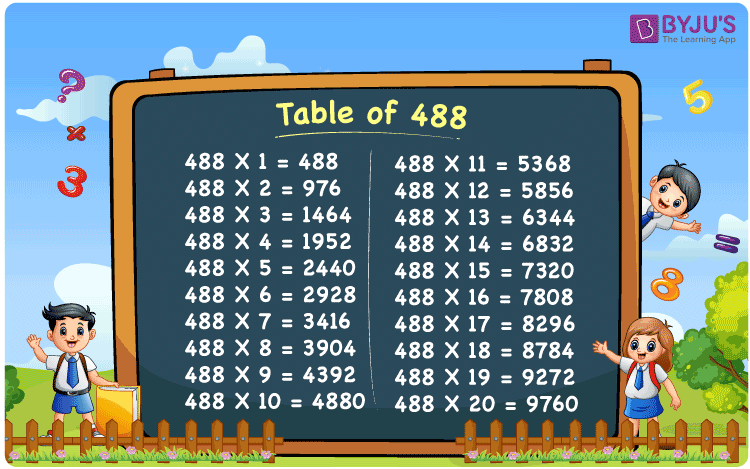Checkout JEE MAINS 2022 Question Paper Analysis : Checkout JEE MAINS 2022 Question Paper Analysis :

# Table of 488

The Multiplication Table of 488 is nothing more than the repetitive addition of the number 488. 488 is an even number that is divisible by 2. Note that 488 is also divisible by 4. Observe that all the multiples of 488 are also divisible by 2 and 4. You can read more on Divisibility rules.

## Table of 488 Chart## What is the 488 Times Table?

In the table of 488, we repeatedly add 488 multiple times. And the table below gives a repetitive addition 10 times for the number 488.

 488 × 1 = 488 488 488 × 2 = 976 488 + 488 = 976 488 × 3 = 1464 488 + 488 + 488 = 1464 488 × 4 = 1952 488 + 488 + 488 + 488 = 1952 488 × 5 = 2440 488 + 488 + 488 + 488 + 488 = 2440 488 × 6 = 2928 488 + 488 + 488 + 488 + 488 + 488 = 2928 488 × 7 = 3416 488 + 488 + 488 + 488 + 488 + 488 + 488 = 3416 488 × 8 = 3904 488 + 488 + 488 + 488 + 488 + 488 + 488 + 488 = 3904 488 × 9 = 4392 488 + 488 + 488 + 488 + 488 + 488 + 488 + 488 + 488 = 4392 488 × 10 = 4880 488 + 488 + 488 + 488 + 488 + 488 + 488 + 488 + 488 + 488 = 4880

## Multiplication Table of 488

Given below is the multiplication table of 488 being done 20 times. The below table helps you with multiplication problems that require the tables of 488.

 488 × 1 = 488 488 × 2 = 976 488 × 3 = 1464 488 × 4 = 1952 488 × 5 = 2440 488 × 6 = 2928 488 × 7 = 3416 488 × 8 = 3904 488 × 9 = 4392 488 × 10 = 4880 488 × 11 = 5368 488 × 12 = 5856 488 × 13 = 6344 488 × 14 = 6832 488 × 15 = 7320 488 × 16 = 7808 488 × 17 = 8296 488 × 18 = 8784 488 × 19 = 9272 488 × 20 = 9760

## Solved Example on Table of 488

Example:

Find the value of y:

y x 20 = 9760.

Solution: y = 488

Given y x 20 = 9760

y = 9760 / 20 = 488.

## Frequently Asked Questions on Table of 488

### What is 18 times 488?

18 times 488 is 8784.

### Is 488 a multiple of 88?

No. 488 is not a multiple of 88.

### Is 488 a prime number?

No. 488 is not a prime number.